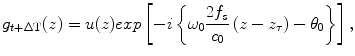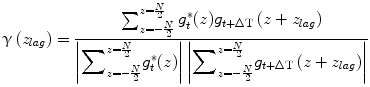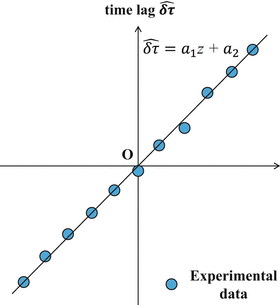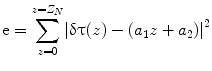# Measurement of Skin Elasticity Using High Frequency Ultrasound Elastography with Intrinsic Deformation Induced by Arterial Pulsation

Fig. 21.1

Block diagram of 3D ultrasound microscope systemFig. 21.2

Schematic of elasticity measurement

### 21.2.2 Subject

Subject is one 24-year-old healthy male. A measurement area is skin in his forearm.

### 21.2.3 Velocity Measurement

The velocity induced by the pulsation was measured from the RF echo data of the fixed position by implementing a 1-D cross correlation method. RF signal of time t at depth z was defined as r t (z). Analytical signal g t (z) was obtained by applying the Hilbert transform to the RF signal r t (z). Pulse waves with angular frequency ω 0 = 2πf 0 were transmitted at a time interval of ΔT. Analytical signal of time t and t + ΔT at depth z can be modeled as(21.1)(21.2)

where u(z) was the envelope of the analytical signal, f s was the sampling frequency, c 0 was the sound speed, θ 0 was the initial phase, and z τ was the true displacement induced by the pulsation. The complex cross-correlation function γ(z lag ) at lag z lag was defined as(21.3)

where N was 1-D cross correlation window of 256 pixels, corresponding to 195 μm depth window with 91 % overlap using hamming window. An index at maximum value of the real part of the Eq. (21.3) corresponded to the index of. In this paper, f s was 1 GHz, and the temporal resolution was enough high to observe the deformation with HFUS of 100 MHz. Additionally, the received RF signal was up-sampled to 4 GHz before implementing the 1-D cross-correlation. Because of these reasons, the estimated displacementwas almost equal to the true displacement z lag . The velocity, denoted by v t + ΔT/2(z), of the skin in his forearm between the interval was given as follows:(21.4)

The acceleration was calculated from the measured velocity. The acceleration was calculated by differentiating the measured velocity as(21.5)

### 21.2.4 Shear Wave Measurement

By comparing an acceleration at reference depth with an acceleration at interest depth, a velocity of shear wave propagating from an artery toward a skin surface can be calculated from a relationship between the depth and the arrival time of shear wave at the depth. The relationship φ(z) can be expressed as(21.6)

where N α was an estimation window of 50 pixels, corresponding to a 50 ms time. A hamming window was used for the estimation. A variable z 0 was a reference depth, and a variable z was an interest depth. A time lag between the reference depth and the interest depth was expressed as follows:(21.7)
The center frequency f c was obtained from the power spectrum calculated by applying the Fourier transform to the acceleration signals. Figure 21.3 shows the relationship between the depth z and the time lag. A regression line can be obtained by applying the least square method to the relationship. A slope a 1 of the regression line can be obtained by minimizing a least mean square error e as follows:Fig. 21.3

Relationship between depth and time lag. Dots: experiments data, and line: regression line calculated by least square method(21.8)
A variable Z N is a total number of the region of interest. The velocity C s of shear wave was given by dividing the depth distance Δz by the estimated slope value